Courses
Courses for Kids
Free study material
Offline Centres
More

# NCERT Solutions for Class 6 Maths Chapter 4 - In HindiLast updated date: 04th Dec 2023
Total views: 429.6k
Views today: 12.29k

## NCERT Solutions for Class 6 Maths Chapter 4 Basic Geometrical Ideas in Hindi PDF Download

Download the Class 6 Maths NCERT Solutions in Hindi medium and English medium as well offered by the leading e-learning platform Vedantu. If you are a student of Class 6, you have reached the right platform. The NCERT Solutions for Class 6 Maths in Hindi provided by us are designed in a simple, straightforward language, which are easy to memorise. You will also be able to download the PDF file for NCERT Solutions for Class 6 Maths in English and Hindi from our website at absolutely free of cost. You can also register Online for NCERT Solutions Class 6 Science tuition on Vedantu.com to score more marks in your examination.

NCERT, which stands for The National Council of Educational Research and Training, is responsible for designing and publishing textbooks for all the classes and subjects. NCERT textbooks covered all the topics and are applicable to the Central Board of Secondary Education (CBSE) and various state boards.

We, at Vedantu, offer free NCERT Solutions in English medium and Hindi medium for all the classes as well. Created by subject matter experts, these NCERT Solutions in Hindi are very helpful to the students of all classes.

## Access NCERT Solution for Hindi Chapter 4- ज्यामितीय अवधारणाएँ

ावली 4.1

1: संलग्न आकृति का प्रयोग करके, निम्न के नाम लिखिए: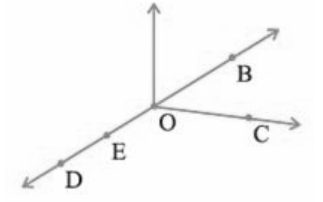1. पाँच बिंदु

2. एक रेखा

3. चार किरणें

4. पाँच रेखाखंड

उत्तर:$\text{1}\text{. }B,\text{ }C,\text{ }O,\text{ }D,\text{ }E,\text{ } \\$

$\text{2}\text{. }\overleftrightarrow{DB}, \\$

$\text{3}\text{. }\overrightarrow{OB},\text{ }\overrightarrow{OC},\text{ }\overrightarrow{OD},\text{ }\overrightarrow{ED}\text{ } \\$

$4.\text{ }\overrightarrow{OB},\text{ }\overrightarrow{OC},\text{ }\overrightarrow{OE},\text{ }\overrightarrow{OD},\text{ }\overrightarrow{ED} \\$

2: संलग्न आकृति में दी हुई रेखा के सभी संभव प्रकारों के नाम लिखिए। आप इन चार बिंदुओं में से किसी भी बिंदु का प्रयोग कर सकते हैं।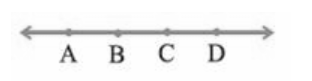उत्तर: $\overleftrightarrow{AB},\overleftrightarrow{BC},\overleftrightarrow{CD,}\overleftrightarrow{BA},\overleftrightarrow{CB},\overleftrightarrow{DC},\overleftrightarrow{AD},\overleftrightarrow{DA},\overleftrightarrow{AC},\overleftrightarrow{CA},\overleftrightarrow{BD},\overleftrightarrow{DB}$

3: संलग्न आकृति को देखकर नाम लिखिए: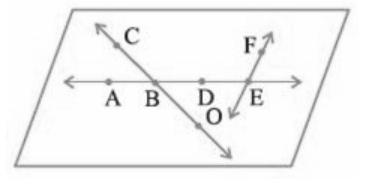1. रेखाएँ जिसमें बिंदु $E$ सम्मिलित हैं

2. $A$ से होकर जाने वाली रेखा

3. वह रेखा जिस पर $O$ स्थित है

4. प्रतिच्छेदी रेखाओं के दो युग्म

उत्तर:  $\left( 1 \right)\text{ }\overleftrightarrow{AE},\text{ }\overleftrightarrow{BE},\text{ }\overleftrightarrow{DE},\text{ }\overleftrightarrow{FE},\text{ } \\$

$\left( 2 \right)\text{ }\overleftrightarrow{AB},\text{ }\overleftrightarrow{AD},\text{ }\overleftrightarrow{AE},\text{ } \\$

$\left( 3 \right)\text{ }\overleftrightarrow{CO},\text{ } \\$

$\left( 4 \right)\text{ }\overleftrightarrow{OC},\text{ }\!\!\And\!\!\text{ }\overleftrightarrow{AE}\text{ } \\$

4: निम्नलिखित से होकर कितनी रेखाएँ खींची जा सकती हैं?

1. एक बिंदु

2. दो बिंदु

उत्तर: (1)एक बिंदु से अनेक रेखा खिची जा सकती है ।

(2) दो बिंदु से एक रेखा खिची जा सकती है ।

5: निम्नलिखित स्थितियों मे से प्रत्येक के लिए एक रफ(Rough) आकृति बनाइए और उचित रूप से उसे नामांकित कीजिए:

1. बिंदु $P$ रेखाखंड $AB$ पर स्थित है।

2. रेखाएँ $XY$ और $PQ$ बिंदु $M$ पर प्रतिच्छेद करती हैं।

3. रेखाएँ $l$ पर $E$ और $F$ स्थित हैं, परंतु $D$ स्थित नहीं है।

4. $OP$ और $OQ$ बिंदु $O$ पर स्थित है।

उत्तर: रफ आकृति-

1)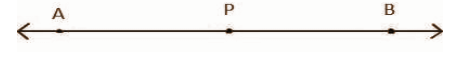2)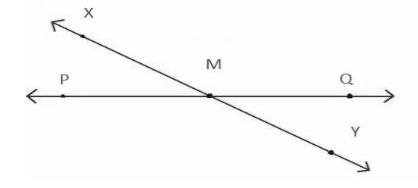3)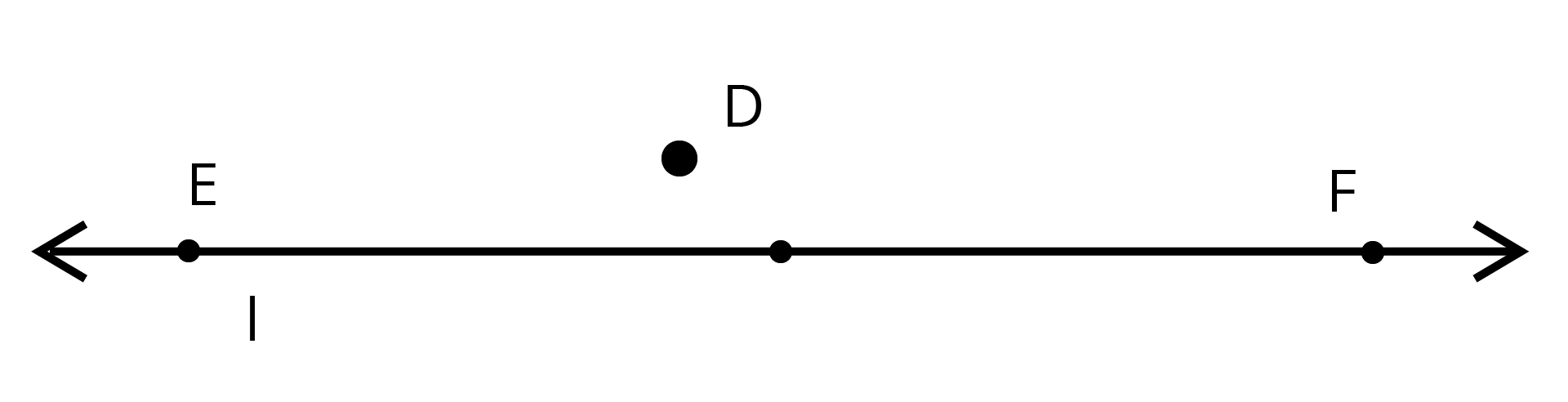4)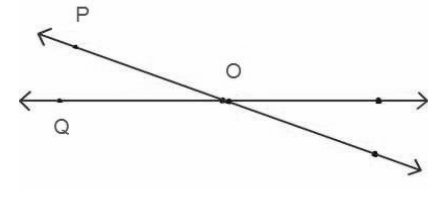6: रेखा $\underline{\mathbf{MN}}$ की संलग्न आकृति को देखिए। इस आकृति के संदर्भ में बताइए कि निम्नलिखित कथन सत्य है या असत्य: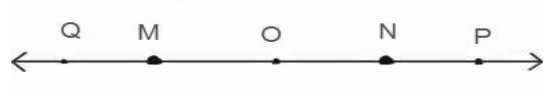1. $Q,\text{ }M,\text{ }O,\text{ }N$ और $P$ रेखा $\underline{\mathbf{MN}}$पर स्थित बिंदु हैं।

2. $M,\text{ }O$ और $N$ रेखाखंड $\underline{\mathbf{MN}}$ पर स्थित बिंदु हैं।

3. $M$ और $N$ रेखाखंड $\underline{\mathbf{MN}}$ के अंत बिंदु हैं।

4. $M$ और $N$ रेखाखंड $\underline{\mathbf{MN}}$ के अंत बिंदु हैं।

5. $M$ रेखाखंड $\underline{QO}$ के दोनों अंत बिंदुओं में से एक बिंदु है।

6. $M$ किरण $\overrightarrow{OP}$ पर एक बिंदु है।

7. किरण $\overrightarrow{OP}$ किरण $\overrightarrow{OP}$ से भिन्न है।

8. किरण $\overrightarrow{OP}$ वही है जो किरण $\overrightarrow{OM}$ है।

9. किरण $\overrightarrow{OM}$ किरण $\overrightarrow{OP}$ के विपरीत(opposite) नहीं है।

10. $O$किरण $\overrightarrow{OP}$ के प्रारम्भिक बिंदु नहीं है।

11. $N$किरण  और $\underline{NM}$ के प्रारम्भिक बिंदु है।

उत्तर: (1) सत्य, (2)सत्य, (3) सत्य, (4) असत्य, (5) असत्य, (6) असत्य, (7) सत्य, (8) असत्य, (9) असत्य, (10) असत्य, (11) सत्य

ावली 4.2

1: नीचे दी हुई वक्रों $(\mathbf{i})$खुली या $(\mathbf{ii})$बंद वक्रों के रूप में वर्गीकृत कीजिए:

(a)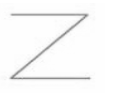(b)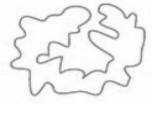(c)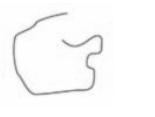(d)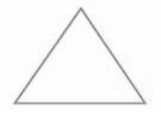(e)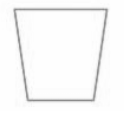उत्तर: (a) खुला, (b) बंद, (c) खुला, (d) बंद, (e) बंद

2: निम्न को स्पष्ट करने के लिए रफ आकृतियाँ बनाइए:

1. खुला वक्र

2. बंद वक्र

उत्तर:

(1)(2)3: कोई भी बहुभुज खींचिए और उसके अभ्यंतर को छायांकित(shade) कीजिए।

उत्तर: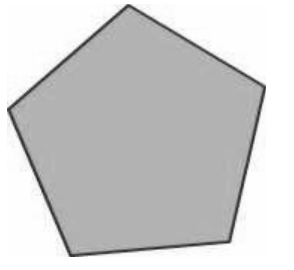4: संलग्न आकृति को देखकर निम्न ों के उत्तर दीजिए।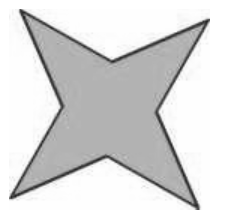1. क्या यह एक वक्र है?

2. क्या यह बंद है?

उत्तर:

1.  हाँ, यह एक वक्र है।

2.  हाँ, यह बंद है।

5: रफ आकृतियाँ बनाकर, यदि सम्भव हो तो निम्न को स्पष्ट कीजिए:

1. एक बंद वक्र जो बहुभुज नहीं है।

2. केवल रेखाखंडों से बनी हुई खुली वक्र

3. दो भुजाओं वाला एक बहुभुज

उत्तर:

1. एक बंद वक्र जो बहुभुज नहीं है।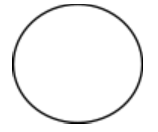1. केवल रेखाखंडों से बनी हुई खुली वक्र।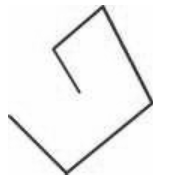1. दो भुजाओं वाला एक बहुभुज संभव नहीं है।

ावली 4.3

1: निचे दी आकृति में, कोणों के नाम लिखिए: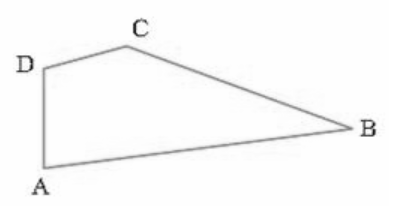उत्तर: आकृति में, कोणों के नाम,

$\angle ADC,\angle DCB,\angle CBA,\angle DAB$

2: संलग्न आकृति में, वे बिंदु लिखिए जो: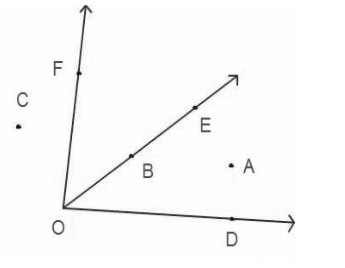1. DOE के अभ्यंतर में स्थित हैं।

2. EOF के बहिर्भाग में स्थित है।

3. EOF पर स्थित है।

उत्तर $\left( 1 \right)\text{ }A,\text{ }\left( 2 \right)\text{ }C,A,D\text{ }\left( 3 \right)\text{ }B$

3: दो कोणों की रफ आकृतियाँ खींचिए जिससे

1. उसमें एक बिंदु उभयनिष्ट हो।

2. उसमें दो बिंदु उभयनिष्ट हों।

3. उसमें तीन बिंदु उभयनिष्ट हो।

4. उसमें चार बिंदु उभयनिष्ट हो।

5. उसमें एक किरण उभयनिष्ट हो।

उत्तर: दो कोणों की रफ आकृतियाँ-

1. उसमें एक बिंदु उभयनिष्ट हो।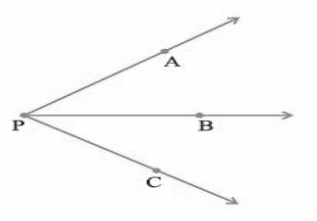1. उसमें दो बिंदु उभयनिष्ट हों।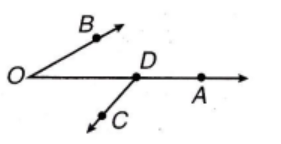1. उसमें तीन बिंदु उभयनिष्ट हो।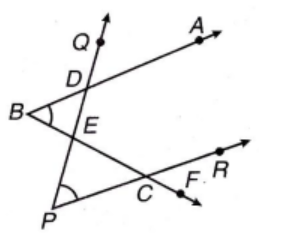1. उसमें चार बिंदु उभयनिष्ट हो।1. उसमें एक किरण उभयनिष्ट हो।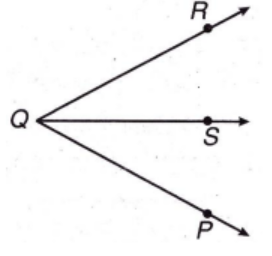ावली 4.4

1: त्रिभुज $\mathbf{ABC}$ का एक रफ चित्र खींचिए। इस त्रिभुज के अभ्यंतर में एक बिंदु $\mathbf{P}$ अंकित कीजिए और उसके बहिर्भाग में एक बिंदु $\mathbf{Q}$ अंकित कीजिए। बिंदु $\mathbf{A}$ इसके अभ्यंतर में स्थित है या बहिर्भाग में स्थित है?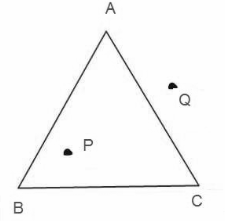उत्तर: बिंदु $A$ त्रिभुज के शीर्ष पर स्थित है।

2: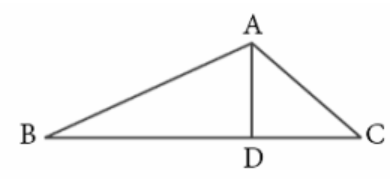1. संलग्न आकृति में तीन त्रिभुजों की पहचान कीजिए।

2. सात कोणों के नाम लिखिए।

3. इसी आकृति में छ: रेखाखंडों के नाम लिखिए।

4. किन दो त्रिभुजों में कोण$\mathbf{B}$ उभयनिष्ट है?

उत्तर: $\left( 1 \right)\text{ }\Delta \text{ }ABC,\text{ }\Delta \text{ }ADC,\text{ }\Delta \text{ }ABD,$

$\left( 2 \right)\text{ }\angle ABC,\text{ }\angle ADB,\text{ }\angle ADC,\text{ }\angle \text{A}CB,\text{ }\angle BAD,\text{ }\angle \text{CA}D,\angle BAC$,

$(3)\underline{AB},\underline{BC},\underline{CA},\underline{AD},\underline{BD},\underline{CD}$

$\left( 4 \right)\text{ }\Delta \text{ }ABC,\text{ }\Delta \text{ }ABD$

ावली 4.5

1: चतुर्भुज $\mathbf{PQRS}$ का एक रफ चित्र खींचिए। इसके विकर्ण खींचिए। इनके नाम लिखिए। क्या विकर्णों का प्रतिच्छेद बिंदु चतुर्भुज के अभ्यंतर में स्थित है या बहिर्भाग में स्थित है?

उत्तर: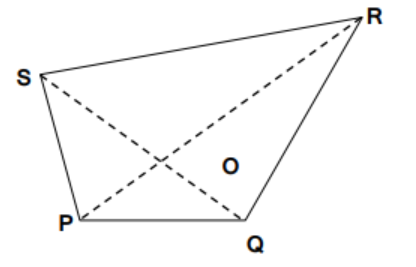विकर्ण $PR$ और $SQ$ विकर्ण एक दूसरे को बिंदु पर प्रतिछेद करते हैं जोकि चतुर्भुज के अभ्यंतर मे स्थित है।

2: चतुर्भुज $\mathbf{ABCD}$ का एक रफ चित्र खींचिए। बताइए:

1. सम्मुख भुजाओं के दो युग्म

2. सम्मुख कोणों के दो युग्म

3. आसन्न भुजाओं के दो युग्म

4. आसन्न कोणों के दो युग्म

उत्तर: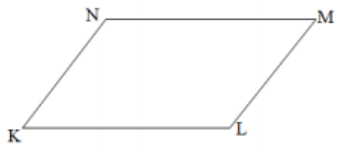1. $KL$ और $MN,\text{ }KN$ और $LM$

2. $\angle K$ और $\angle M,\angle L$ और $\angle N$

3. $KN$ और $NM\text{ },\text{ }KL$ और $LM$

4.   $\angle K$ और $\angle N\text{ },\angle L$ और $\angle M$

3: पट्टियाँ और उन्हें बांधने की वस्तुएँ लेकर एक त्रिभुज बनाइए। त्रिभुज के किसी एक शीर्ष पर पट्टियों को अंदर की ओर दबाने का प्रयत्न कीजिए। यही कार्य चतुर्भुज के लिए भी कीजिए। क्या त्रिभुज में कोई परिवर्तन आया? क्या चतुर्भुज में कोई परिवर्तन हुआ? क्या त्रिभुज एक दृढ़ आकृति है? क्या कारण है कि विद्युत टावरों(electric towers) जैसी संरचनाओं में त्रिभुज आकारों का प्रयोग किया जाता है, चतुर्भुजीय आकारों का नहीं?

उत्तर: चतुर्भुज की तुलना में त्रिभुज एक दृढ़ आकृति है। इसलिए त्रिभुज के किसी भी शीर्ष पर दबाने से उसके आकार में कोई विकृति नहीं आती है। यही कारण है कि विद्युत टावरों जैसी संरचनाओं में त्रिभुज के आकार का प्रयोग किया जाता है, चतुर्भुजीय आकारों का नहीं।

ावली 4.6

1.संलग्न आकृति देखकर लिखिए: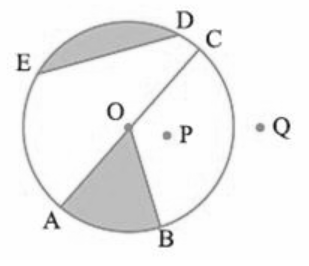1. वृत का केंद्र

2. तीन त्रिज्याएँ

3. एक व्यास

4. एक जीवा

5. अभ्यंतर में दो बिंदु

6. बहिर्भाग में एक बिंदु

7. एक त्रिज्यखंड

8. एक वृतखंड

उत्तर:

1. वृत का केंद्र-O

2. तीन त्रिज्याएँ$-OA,OB,OC$

3. एक व्यास$-AC$

4. एक जीवा$-ED$

5. अभ्यंतर में दो बिंदु$-O,\text{ }P$

6. बहिर्भाग में एक बिंदु$-Q$

7. एक त्रिज्यखंड$-OAB$

8. एक वृतखंड $\text{ }ED$

2.

1. क्या वृत का प्रत्येक व्यास उसकी एक जीवा भी होता है?

2.  क्या वृत का प्रत्येक जीवा उसका एक व्यास भी होती है?

उत्तर:

1. हाँ, वृत का प्रत्येक व्यास उसकी एक जीवा भी होता है।

2. नहीं,वृत का प्रत्येक जीवा उसका एक व्यास भी होती है।

3: कोई वृत खींचिए और निम्न को अंकित कीजिए:

1. उसका केंद्र

2. एक वृतखंड

3. एक त्रिज्या

4. उसके अभ्यंतर में एक बिंदु

5. एक व्यास

6. उसके बहिर्भाग में एक बिंदु

7. एक त्रिज्यखंड $(\mathbf{h})$एक चाप

उत्तर:1. उसका केंद्र $-\text{ }O$

2. एक वृतखंड $-\text{ }OA$

3. एक त्रिज्या $-\text{ }AB$

4. उसके अभ्यंतर में एक बिंदु $-\text{ }P$

5. एक व्यास $-\text{ }AC$

6. उसके बहिर्भाग में एक बिंदु $-\text{ }Q$

7. एक त्रिज्यखंड$-OBC$

8. एक चाप $-\text{ BC}$

4: सत्य या असत्य बताइए:

1. वृत के दो व्यास अवश्य ही प्रतिच्छेद करेंगे।

2. वृत का केंद्र सदैव उसके अभ्यंतर में स्थित होता है।

उत्तर: (1) सत्य, (2) सत्य

## NCERT Solutions for Class 6 Maths Chapter 4 Basic Geometrical Ideas in Hindi

Chapter-wise NCERT Solutions are provided everywhere on the internet with an aim to help the students to gain a comprehensive understanding. Class 6 Maths Chapter 4 solution Hindi mediums are created by our in-house experts keeping the understanding ability of all types of candidates in mind. NCERT textbooks and solutions are built to give a strong foundation to every concept. These NCERT Solutions for Class 6 Maths Chapter 4 in Hindi ensure a smooth understanding of all the concepts including the advanced concepts covered in the textbook.

NCERT Solutions in Hindi medium have been created keeping those students in mind who are studying in a Hindi medium school. These NCERT Solutions for Class 6 Maths Basic Geometrical Ideas in Hindi medium pdf download have innumerable benefits as these are created in simple and easy-to-understand language. The best feature of these solutions is a free download option. Students of Class 6 can download these solutions at any time as per their convenience for self-study purpose.

These solutions are nothing but a compilation of all the answers to the questions of the textbook exercises. The answers/ solutions are given in a stepwise format and very well researched by the subject matter experts who have relevant experience in this field. Relevant diagrams, graphs, illustrations are provided along with the answers wherever required. In nutshell, NCERT Solutions for Class 6 Maths in Hindi come really handy in exam preparation and quick revision as well prior to the final examinations.

## FAQs on NCERT Solutions for Class 6 Maths Chapter 4 - In Hindi

Q1. Is it required to solve each and every problem of NCERT Solutions for Chapter 4 of Class 6 Maths?

It is required to solve each and every problem of NCERT Solutions for Chapter 4 of Class 6 Maths if you want to get high marks in the exam because the majority of the questions are asked from NCERT exercises only. If you go through the NCERT Solutions of each chapter of your Maths NCERT and practice them again and again, you will surely be able to ace the exam well. The NCERT Solutions for Chapter 4 of Class 6 Maths are easily available on the Vedantu website and the Vedantu app free of cost.

Q2. Where can I find the complete solutions of NCERT Chapter 4 Class 6?

You can find the complete solutions of NCERT Chapter 4 Class 6 on the official website of Vedantu. All the solutions are provided by expert teachers and are explained in simple ways. You can download them in pdf formats too so that you can read them without the internet. Going through the solutions of NCERT exercises should be a part of your Class 6 Maths exam preparation.

Q3. How many chapters are there in the CBSE Class 6 Maths apart from Chapter 4?

Total 14 chapters are there in the CBSE Class 6 Maths from four different portions: arithmetic, algebra, mensuration, and geometry. They are namely, Knowing Our Numbers, Whole Numbers, Playing With Numbers, Basic Geometrical Ideas, Understanding Elementary Shapes, Integers, Fractions, Decimals, Mensuration, Algebra, Data Handling, Ratio and Proportion, Symmetry and Practical Geometry. You can download the PDF of CBSE Class 6 Maths from Vedantu to go through them offline.

Q4. From where can I download the NCERT Solutions for Chapter 4 of Class 6 Maths?

You can download the NCERT Class 6 Maths Chapter 4 from the website of Vedantu in PDF format to read it without the internet at any time, sitting anywhere, without spending a single penny. Vedantu also provides you with the detailed solution of each chapter of all the subjects for free. You can download the solution in PDF format or go through them from the website itself.

Q5. How to download NCERT Solutions for Chapter 4 of Class 6 Maths in Hindi?

To download the NCERT Solutions for Chapter 4 of Class 6 Maths in Hindi, you need to visit the official website of Vedantu. Here, you will find the links to the NCERT books for each subject in both English and Hindi, taught in Class 6. On opening each link, you will find the links to download the pdf of that particular book. Here you will get the PDFs of chapter-wise solutions, sample papers, and worksheets too.